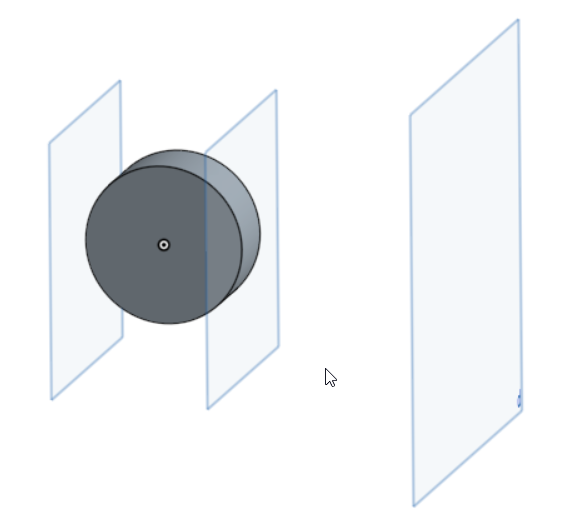Welcome to the Onshape forum! Ask questions and join in the discussions about everything Onshape.

First time visiting? Here are some places to start:

1. Looking for a certain topic? Check out the categories filter or use Search (upper right).
2. Need support? Ask a question to our Community Support category.
3. Please submit support tickets for bugs but you can request improvements in the Product Feedback category.
4. Be respectful, on topic and if you see a problem, Flag it.

If you would like to contact our Community Manager personally, feel free to send a private message or an email.

# How to find extent planes for a solid in a specific direction

edited August 2017
I have created a feature script feature. The user can select

• S is Solid
• P is Plane
I would like to generate two planes that bound in the direction of normal to P

Before: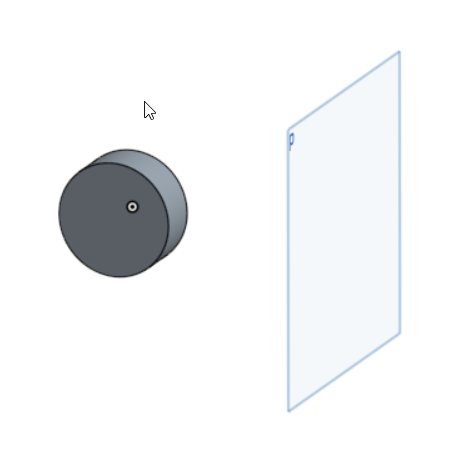After: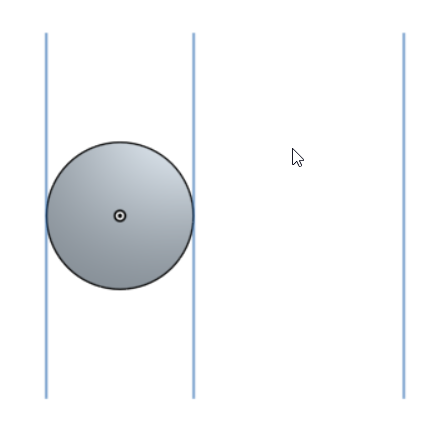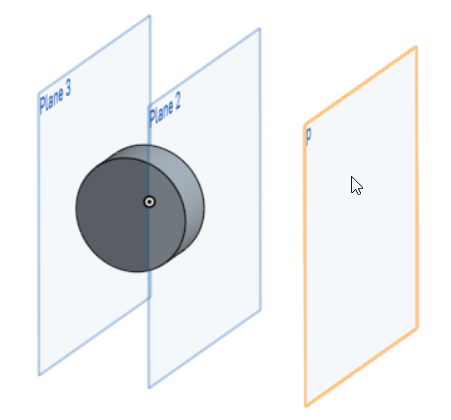Any ideas how to do this?

Tagged:

• edited August 2017
evBox3d of solid S with `tight` option will give you 2 vectors and the direction of plane P will give you the normal, so you can construct 2 planes.
in the arbitary case you may requare to construct local CS to pass into evBox3d, as X axis could be used normal of plane P, Z vector can be obtained by perpendicularVector(xVector)

• Here is a solution

FeatureScript 660;
import(path : "onshape/std/geometry.fs", version : "660.0");

annotation { "Feature Type Name" : "Auto Rib" }
export const myFeature = defineFeature(function(context is Context, id is Id, definition is map)
precondition
{
annotation { "Name" : "Part", "Filter" : BodyType.SOLID, "MaxNumberOfPicks" : 1 }
definition.Part is Query;

annotation { "Name" : "Rib Direction", "Filter" : GeometryType.PLANE, "MaxNumberOfPicks" : 1 }
definition.Plane is Query;

annotation { "Name" : "Number of Ribs" }
isInteger(definition.myCount, POSITIVE_COUNT_BOUNDS);

annotation { "Name" : "Rib Thickness" }
isLength(definition.myLength, LENGTH_BOUNDS);

}
{
const p is Plane = evPlane(context, {
"face" : definition.Plane
});

var b is Box3d = evBox3d(context, {
"topology" : definition.Part,
"cSys" : coordSystem(p)
});

const t is Transform = toWorld(coordSystem(p));

b = box3d(t * b.minCorner, t*b.maxCorner);

opPoint(context, id + "minCorner", {
"point" : b.minCorner
});

opPoint(context, id + "maxCorner", {
"point" : b.maxCorner
});

const p0 = dummyQuery(id + "minCorner", EntityType.VERTEX);
const p1 = dummyQuery(id + "maxCorner", EntityType.VERTEX);

cPlane(context,
id + "pl0",
{ "entities" : qUnion([definition.Plane, p0]),
"cplaneType" : CPlaneType.PLANE_POINT,

});

cPlane(context,
id + "pl1",
{ "entities" : qUnion([definition.Plane, p1]),
"cplaneType" : CPlaneType.PLANE_POINT,

});

});

The test result is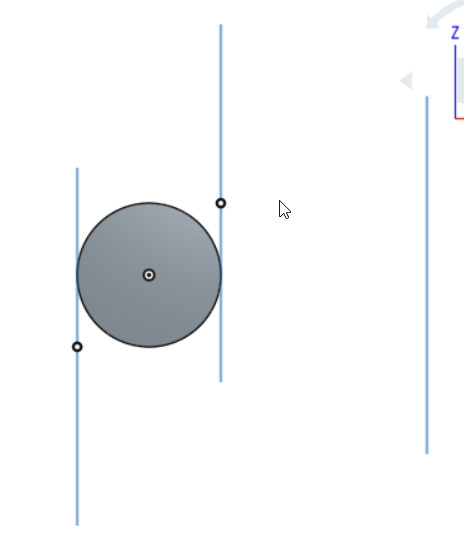I would prefer the the planes are vertically aligned correctly but the result is not incorrect.
• edited August 2017
i see here some redundant code, when you obtained "p" you can make construction planes in a fewer steps like
p = coordSystem(p);
b = evBox3d(context, {
"topology" : definition.Part,
"cSys" : p
});

b.minCorner = toWorld(p,b.minCorner);
b.maxCorner = toWorld(p,b.maxCorner);

opPlane(context, id+"minPlane",{
"plane": plane(b.minCorner, p.zAxis),
.....
});

opPlane(context, id+"maxPlane",{
"plane": plane(b.maxCorner, p.zAxis),
.....
});
• A better solution is to project the box3d corners onto the original plane vector. Then the result planes are just offset from the original

FeatureScript 660;
import(path : "onshape/std/geometry.fs", version : "660.0");

annotation { "Feature Type Name" : "Auto Rib" }
export const myFeature = defineFeature(function(context is Context, id is Id, definition is map)
precondition
{
annotation { "Name" : "Part", "Filter" : BodyType.SOLID, "MaxNumberOfPicks" : 1 }
definition.Part is Query;

annotation { "Name" : "Rib Direction", "Filter" : GeometryType.PLANE, "MaxNumberOfPicks" : 1 }
definition.Plane is Query;

annotation { "Name" : "Number of Ribs" }
isInteger(definition.myCount, POSITIVE_COUNT_BOUNDS);

annotation { "Name" : "Rib Thickness" }
isLength(definition.myLength, LENGTH_BOUNDS);

}
{
const p is Plane = evPlane(context, {
"face" : definition.Plane
});

var b is Box3d = evBox3d(context, {
"topology" : definition.Part,
"cSys" : coordSystem(p)
});

const t is Transform = toWorld(coordSystem(p));

b = box3d(t * b.minCorner, t * b.maxCorner);

const line is Line = line(p.origin, p.normal);

const p0 is Vector = project(line, b.minCorner);
const p1 is Vector = project(line, b.maxCorner);

opPoint(context, id + "p0", {
"point" : p0
});

opPoint(context, id + "p1", {
"point" : p1
});

const p0Id = dummyQuery(id + "p0", EntityType.VERTEX);
const p1Id = dummyQuery(id + "p1", EntityType.VERTEX);

cPlane(context,
id + "pl0",
{ "entities" : qUnion([definition.Plane, p0Id]),
"cplaneType" : CPlaneType.PLANE_POINT,

});

cPlane(context,
id + "pl1",
{ "entities" : qUnion([definition.Plane, p1Id]),
"cplaneType" : CPlaneType.PLANE_POINT,

});

});

• And dropping down to using opPlane instead of cPlane makes the code shorter and avoid inserting dummy points into the model

FeatureScript 660;
import(path : "onshape/std/geometry.fs", version : "660.0");

annotation { "Feature Type Name" : "Auto Rib" }
export const myFeature = defineFeature(function(context is Context, id is Id, definition is map)
precondition
{
annotation { "Name" : "Part", "Filter" : BodyType.SOLID, "MaxNumberOfPicks" : 1 }
definition.Part is Query;

annotation { "Name" : "Rib Direction", "Filter" : GeometryType.PLANE, "MaxNumberOfPicks" : 1 }
definition.Plane is Query;

annotation { "Name" : "Number of Ribs" }
isInteger(definition.myCount, POSITIVE_COUNT_BOUNDS);

annotation { "Name" : "Rib Thickness" }
isLength(definition.myLength, LENGTH_BOUNDS);

}
{
const p is Plane = evPlane(context, {
"face" : definition.Plane
});

var b is Box3d = evBox3d(context, {
"topology" : definition.Part,
"cSys" : coordSystem(p)
});

const t is Transform = toWorld(coordSystem(p));

b = box3d(t * b.minCorner, t * b.maxCorner);

const line is Line = line(p.origin, p.normal);

const p0 is Vector = project(line, b.minCorner);
const p1 is Vector = project(line, b.maxCorner);

const pl0 is Plane = plane(p0, p.normal);
const pl1 is Plane = plane(p1, p.normal);

opPlane(context, id + "pl0", {
"plane" : pl0,
});

opPlane(context, id + "pl1", {
"plane" : pl1,
});

});